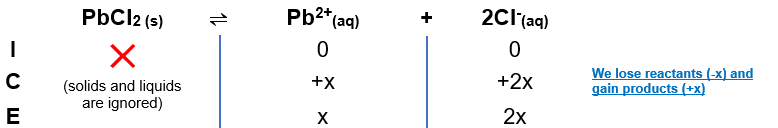# Problem: Please answer this question with a brief explanation.The solubility of lead (II) chloride (PbCl2) is 1.6 x 10-2 M. What is the Ksp of PbCl2? A) 4.1 x 10-6 B) 1.6 x 10-5 C) 5.0 X 10-4 D) 3.1 x 10-7 E) 1.6 x 10-2

###### FREE Expert Solution

Dissociation of PbCl2:

PbCl2(s)   Pb2+(aq) + 2Cl(aq)

ICE tableThe Ksp expression then is:

$\overline{){{\mathbf{K}}}_{{\mathbf{sp}}}{\mathbf{=}}\frac{\mathbf{products}}{\overline{)\mathbf{reactants}}}{\mathbf{=}}{\mathbf{\left[}}{{\mathbf{Pb}}}^{\mathbf{2}\mathbf{+}}{\mathbf{\right]}}{\mathbf{\left[}}{{\mathbf{Cl}}}^{{\mathbf{-}}}{{\mathbf{\right]}}}^{{\mathbf{2}}}}$

81% (4 ratings)###### Problem Details

The solubility of lead (II) chloride (PbCl2) is 1.6 x 10-2 M. What is the Ksp of PbCl2

A) 4.1 x 10-6

B) 1.6 x 10-5

C) 5.0 X 10-4

D) 3.1 x 10-7

E) 1.6 x 10-2